# 直线方程的点斜式

### 直线方程的"点斜"式是：

y − y1 = m(x − x1)

• 直线上的一
• 和 直线的坡度 时，

## 它代表什么？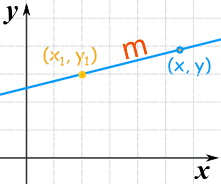(x1, y1)已知

m 是直线的 坡度

(x, y) 是直线上任何其他一点

## 来了解它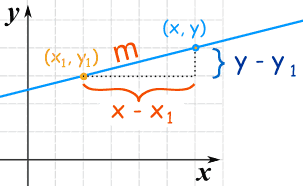从坡度开始： 这样重排：   变成这样：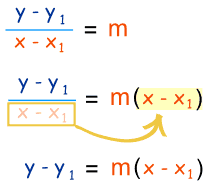### 例子：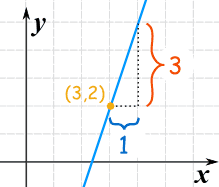y − y1 = m(x − x1)

y − 2 = 3(x − 3)

y − 2 = 3x − 9

y = 3x − 9 + 2

y = 3x − 7

### 例子 2：m = −3 1 = −3

y − y1 = m(x − x1)

y − 0 = −3(x − 0)

y = −3x

### 例子 3: 垂直线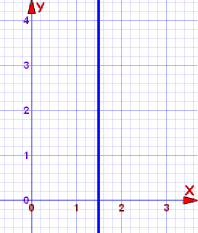x = 1.5

## 用这个 y = mx + b 呢？

"b" 的值（叫Y截距）是直线与Y轴相交的地方。

 开始： y − y1 = m(x − x1) (x1, y1) 其实是 (0, b)： y − b = m(x − 0) 就是： y − b = mx 把 b 换边： y = mx + b